# Worksheet 19 Titration of Polyprotic Acids Name: 1. Calculate the sulfite ion [SO3'] concentration in a...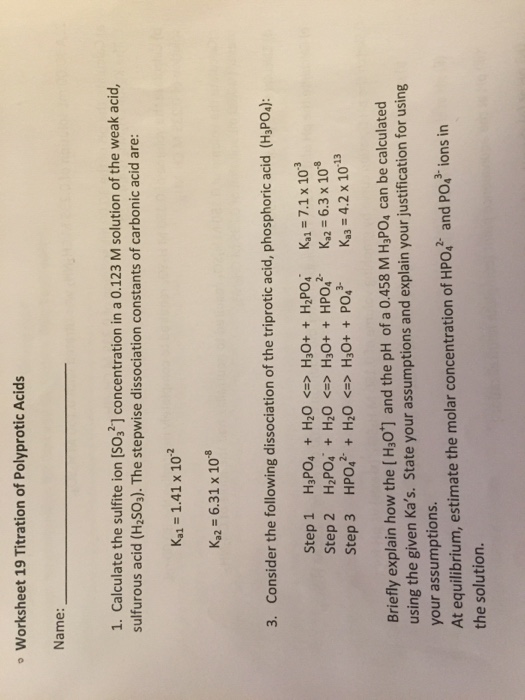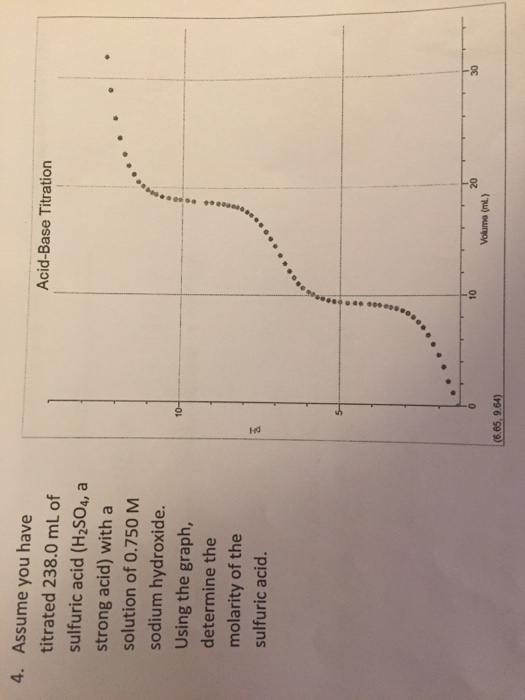Worksheet 19 Titration of Polyprotic Acids Name: 1. Calculate the sulfite ion [SO3'] concentration in a 0.123 M solution of the weak acid, sulfurous acid (H2SO3). The stepwise dissociation constants of carbonic acid are: Ka1 1.41 x 102 Ka2-6.31 x 108 3. Consider the following dissociation of the triprotic acid, phosphoric acid (HsPOs): Step 1 H3POa + H2O <=> H30+ + H2PO4, Step 2 HP0i + H2O <=> H30+ + HP042- Step 3 HPO42-+ H2O <-> H3O+ + PO43- Kal=7.1 x 10-3 Ka2-6.3 x 10-8 Kg = 4.2 x 10-13 Briefly explain how the [Hs0'] and the pH of a 0.458 M H3PO4 can be calculated using the given Ka's. State your assumptions and explain your justification for using your assumptions. At equilibrium, estimate the molar concentration of HPo,2 and PO,3 ions in the solution.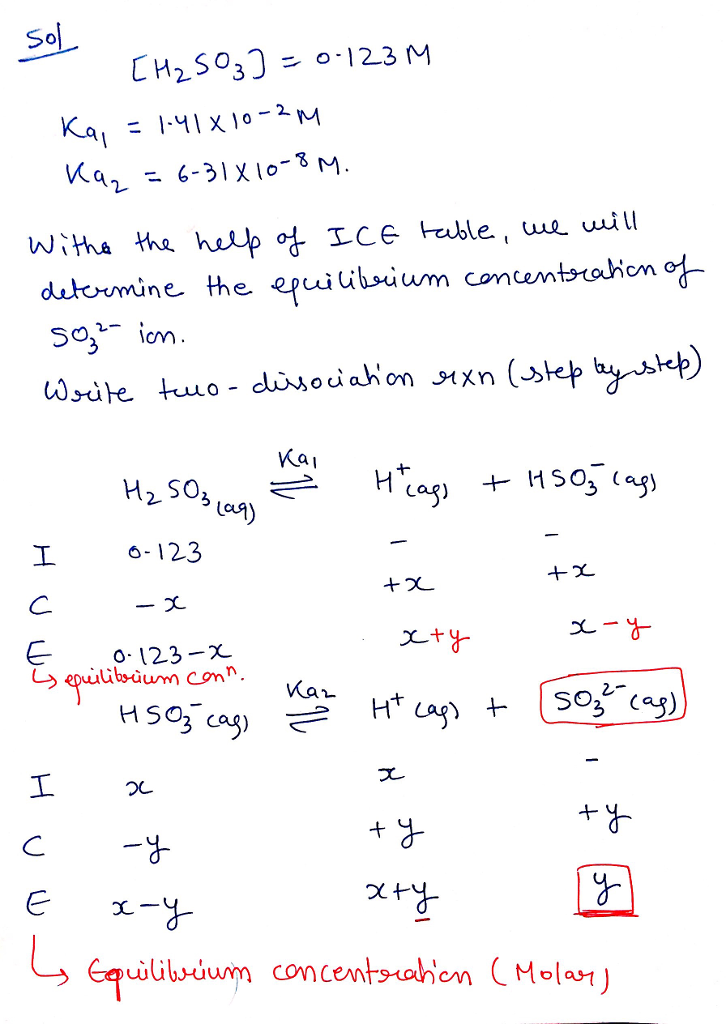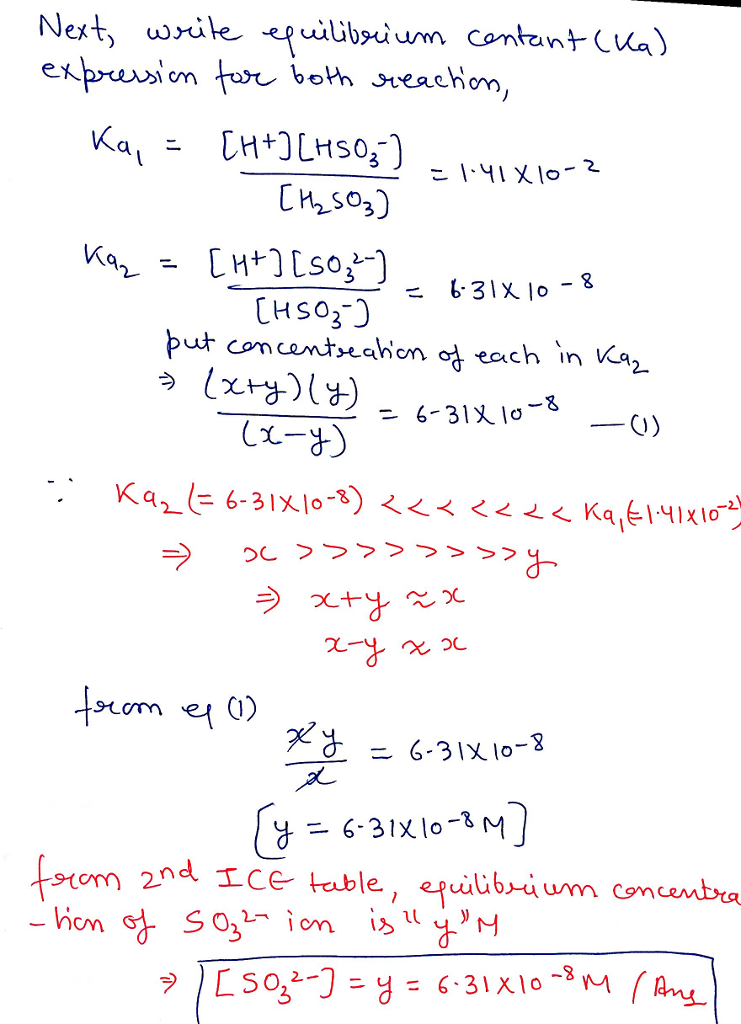#### Earn Coin

Coins can be redeemed for fabulous gifts.

Similar Homework Help Questions
• ### 71% Calculate the pH and concentration of all species in a 027M Na2 SOs (sodium sulfite)...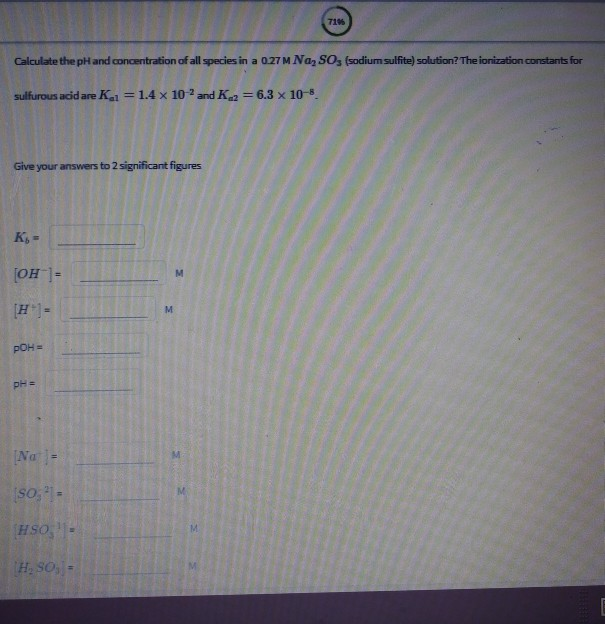71% Calculate the pH and concentration of all species in a 027M Na2 SOs (sodium sulfite) solution? The ionization constants for sulfurous acidare Kal 1.4 x 10-2 and K2 6.3 x 10-8 Give your answers to 2 significant figures OH 1 POH PH- İHS S05 - Estimate the pH and the concentrations of all species in a 0.350 M phasphoric acid solution Answer thisquestion by matching each of the questions (on the left) with the correct value (on the right)...

• ### Polyprotic acids have more than one proton to donate to water, therefore have more than one...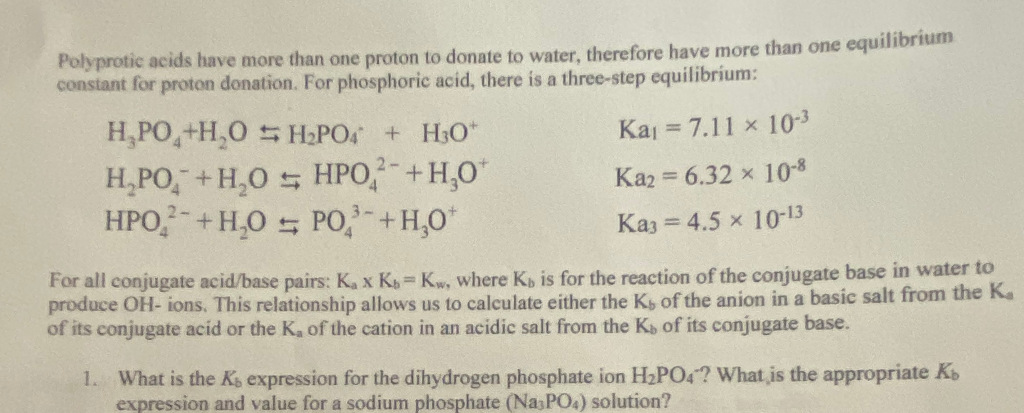Polyprotic acids have more than one proton to donate to water, therefore have more than one equid constant for proton donation. For phosphoric acid, there is a three-step equilibrium: HAPO+H,O SHPO4 + H30+ H,PO," +H2O 5 HPO - +4,0* HPO,- +H,0 5 PO."-+H,0* Kaj = 7.11 10-3 Ka2 = 6.32 x 10-8 Kaj = 4.5 x 10-13 For all conjugate acid/base pairs: K x K K , where K is for the reaction of the conjugate base in water to...